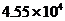Chapter 10, Problem 28QAP### Introductory Chemistry: A Foundati...

9th Edition
Steven S. Zumdahl + 1 other
ISBN: 9781337399425

#### Solutions

Chapter
Section### Introductory Chemistry: A Foundati...

9th Edition
Steven S. Zumdahl + 1 other
ISBN: 9781337399425
Textbook Problem
1 views

# Convert the following numbers of calories or kilocalories into joules or kilojoules. 7845 cal i>cal 62.142 kcal43.024 cal

Interpretation Introduction

(a)

Interpretation:

Convert 7845 cal to joules.

Concept Introduction:

A conversion factor is a numerical ratio which is used to show a measurement in one unit as another unit. It is always equal to 1.

Explanation

The given conversion is: conversion of calories to joules.

1 calorie is equal to the 4.184 joules.

1 cal = 4.184 J

Thus, conversion factor is given by:

4.184 J1 cal

So,

Interpretation Introduction

(b)

Interpretation:

Convert 4.55×104 cal to joules.

Concept Introduction:

A conversion factor is a numerical ratio which is used to show a measurement in one unit as another unit. It is always equal to 1.

Interpretation Introduction

(c)

Interpretation:

Convert 62.142 kcal to kilojoules.

Concept Introduction:

A conversion factor is a numerical ratio which is used to show a measurement in one unit as another unit. It is always equal to 1.

Interpretation Introduction

(d)

Interpretation:

Convert 43, 024 kcal to kilojoules.

Concept Introduction:

A conversion factor is a numerical ratio which is used to show a measurement in one unit as another unit. It is always equal to 1.

### Still sussing out bartleby?

Check out a sample textbook solution.

See a sample solution

#### The Solution to Your Study Problems

Bartleby provides explanations to thousands of textbook problems written by our experts, many with advanced degrees!

Get Started